# Realization of A New Linear System Explaining the Fundamental Laws of Light and Energy in Infinite Spaces

Mohamed Daris*

Department of Physics, Morocco

Received Date: August 25, 2018;  Published Date: December 14, 2018

#### Abstract

In doing this work I try to better understand and understand relativity by giving it another form that better describes the relation between the energy of a particle and the speed of light and I find a new parameter of time that I use it to explain deeper my theorem which is based on this newly exploiting aspect through a development of a special mathematical concept that gave me specific access to develop and bring out the energy theorem. I would also say that the theorem is a new mathematical and physical structure that are both linking and appropriate the speed of light remains the same my concept just explained the phenomena that we can find them in far galaxies or even in our galaxy, as for example the black hole because at the level of these giant physical bodies matter it exists only in the state of emptiness with enormous speed and infinite time. So, I try to give a new concept for this coast.

Keywords: New relationship of energies; New fundamental physics; Speed of light; Fundamental relationship; Universal infinite energy; New time parameter

Abbreviations: C: Speed of Light; E: The Energy of Light; E: New Speed of Light; TF: Final Time Parameter; m: Mass of Matter

#### Introduction

In this study, I put relations energy time and energy speed and material very developed to solve the classic theme of the equation of light and to come to make a new model of the equation of light by the hypothesis of creation of a new time parameter and a new concept which is based on this parameter which is a mathematical equation that I put them to get to make the new linear model of the equation of light in connection with the final time parameter .

Relationship sutured endless new

The following new Relationship that we have: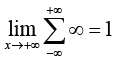Demonstrations were:Or:So, this implies the following relationship:And:Or we have: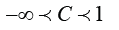This implies that:Or we have:And it was for: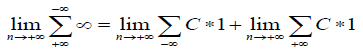So the result is equal to: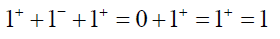So we have the end result is:Direct result of the physical fundamental law: In another demonstration an equation which connect all the universal parameter of the universe between them this equation become:And it is known after the universal relationship:And we: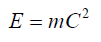So we: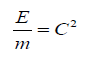Was:So we: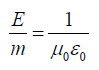So we will:Returning to our equation:

We have: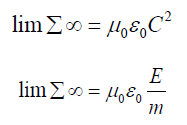And we all at some point the universe tend toward a moment that I called:

TF

So we:So we: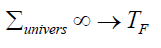With:We have: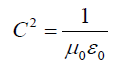And:So we:So we: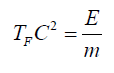We set: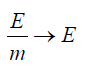So we: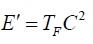And we: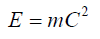So we will:And: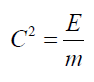So we:So we have the following general relationship:And with:So we:So:And we:So: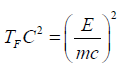So: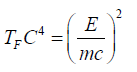So we have the following general fundamental relationship:A relation can be deduced between E and E’: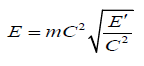So we: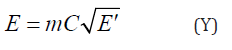Explanation of two theorems (x) and (y) dimension of a physical perspective;

For (x):

I deduced that the time is the universe is relative to the speed and mass of the materiel according to its energy E.

I was always positive because:So we can see that time very high compared to the speed and the materiel itself.

For (y):

We have a new energy that appears after time TF is a universal infinite energy is always positive is proportional to the energy E and it can reach the whole of the universe and explains the total flow of matter through transformation of these two energies.

Note: All these calculations are made in order to correct and retrieve a developed and corrective version of the classic Einstein relation and would be linked to anew time parameter that is TF.

#### Conclusion

In doing this work I try to better understand and understand relativity by giving it another form that better describes the relation between the energy of a particle and the speed of light and I find a new parameter of time that I use it to explain deeper my theorem which is based on this newly exploiting aspect through a development of a special mathematical concept that gave me specific access to develop and bring out the energy theorem.

None

#### Conflict of Interest

No conflict of interest.

Article Details
Citation
Keywords
Scroll to
Scroll to Top